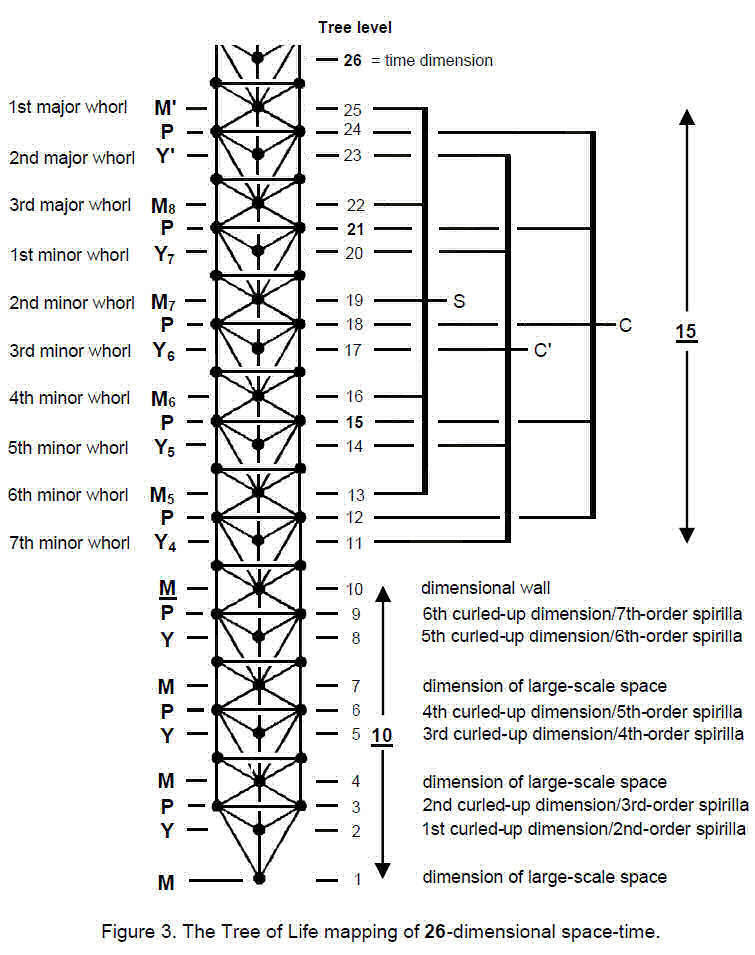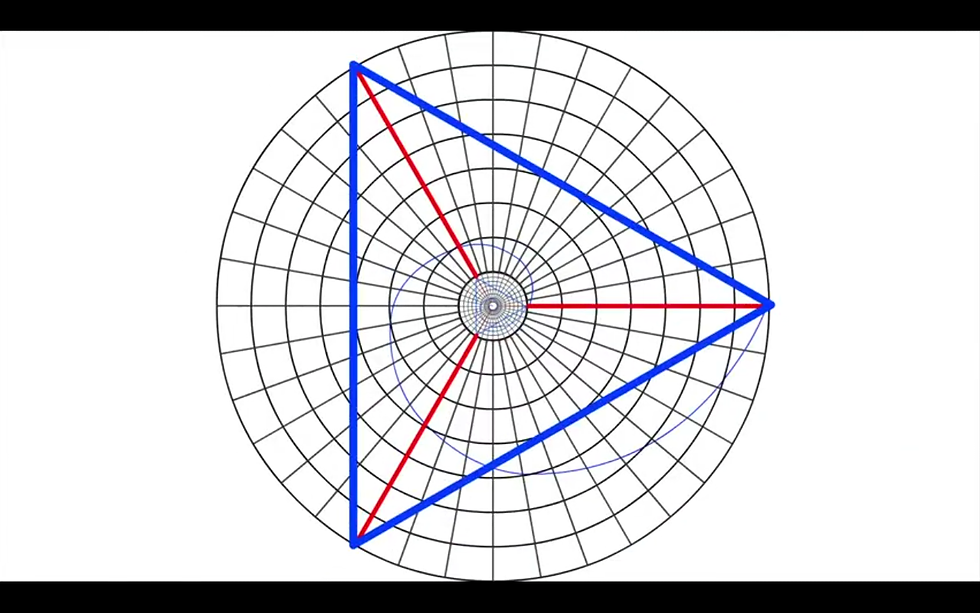top of page
Search

# Cosmic sphere and the 26 superstring dimensions

Updated: Apr 11, 2021

If the circumference of a circle is phi then its radius is 0.2575181074, if the radius of a sphere is 0.2575181074 then its volume is 0.072 and its surface area is 0.83 which is close to the value of 5/6• 0.072 is related to 72 which is the Tetragrammaton/tetractys/tree of life and the infinite tetrahedron grid is made out of tree of life's.

• the radius of the sphere is 0.26 which is related to the number 26 which is related to the trinity 26 superstring dimensions of the 512 tetrahedron grid also the 4th line in the Tetragrammaton which is related to the fine structure constant is equivalent to 26 and corresponds to the tree of life and the 4 worlds.

• the number 0.83 can be related to -1/12 which is the infinite tetractys/infinite tetrahedron grid. this shows that 0.83 or -1/12 encodes the infinite tetrahedron grid like a cosmic egg/cosmic sphere.

The trinity 26 dimensions can form a triangle(which encodes all Harmonics/frequencies/vibrational dimensions) in this circle(Sphere) which is created by a fibonacci spiral:The growth of this spiral is at the angle 137(The golden angle). the spiral also forms the fibonacci numbers which can be a repeating 24 digit number series which adds up to 108(frequency of Om and the frequency that forms the sri yantra/64 tetrahedron grid) and every time this series goes up 4 octaves(432) there is a vibrational dimension(and it forms a 64 tetrahedron grid)